Function Repository Resource:

# EigenvectorPlot

Visualize the Eigenvectors of a 2 x 2 or 3 x 3 matrix

Contributed by: Jon McLoone
 ResourceFunction["EigenvectorPlot"][m] visualizes the Eigenvectors of 2 x 2 or 3 x 3 matrix m.

## Details and Options

For 2 x 2 matrices all options of VectorPlot are supported
For 3 x 3 matrices all options of VectorPlot3D are supported

## Examples

### Basic Examples (2)

For 2 x 2 matrices, the vector field generated by left-multiplying by the matrix is displayed on the unit circle, together with dashed lines indicating the Eigenvectors. It can be observed that the vectors are perpendicular to the unit circle only at the Eigenvector lines:

 In:=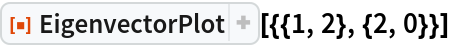Out=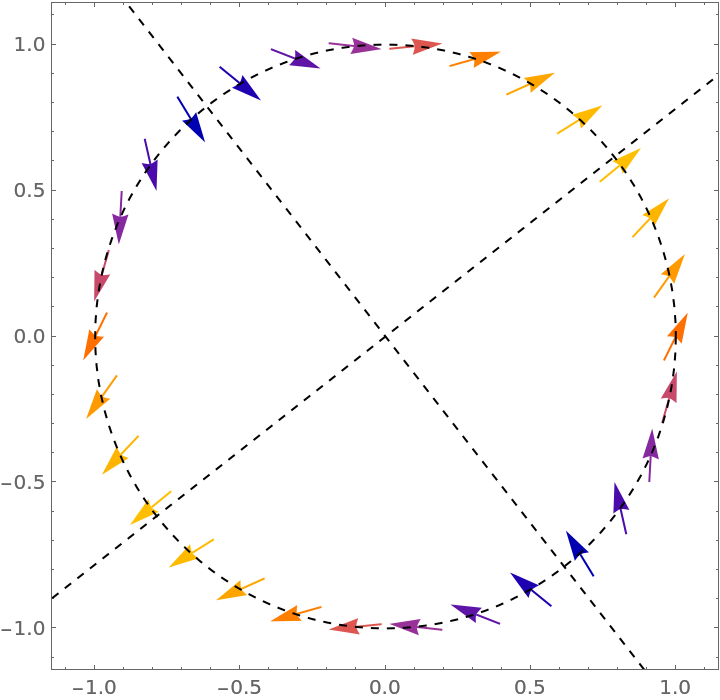For 3 x 3 matrices, the vector field is plotted on the unit sphere:

 In:=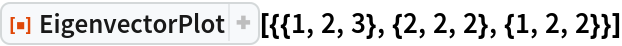Out=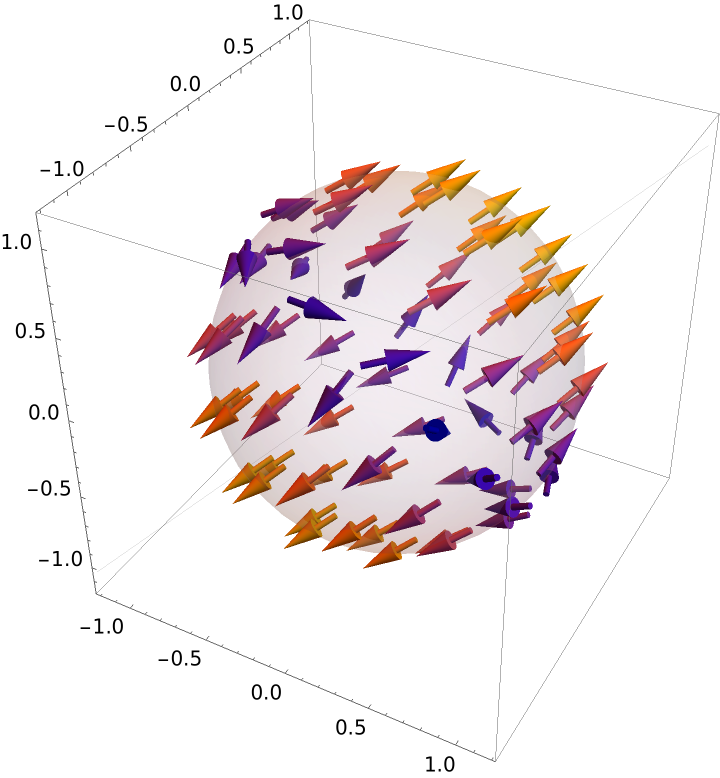### Options (2)

The relevant options for VectorPlot and VectorPlot3D can be used:

 In:=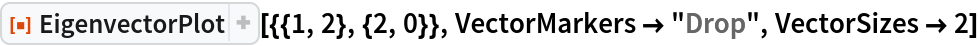Out=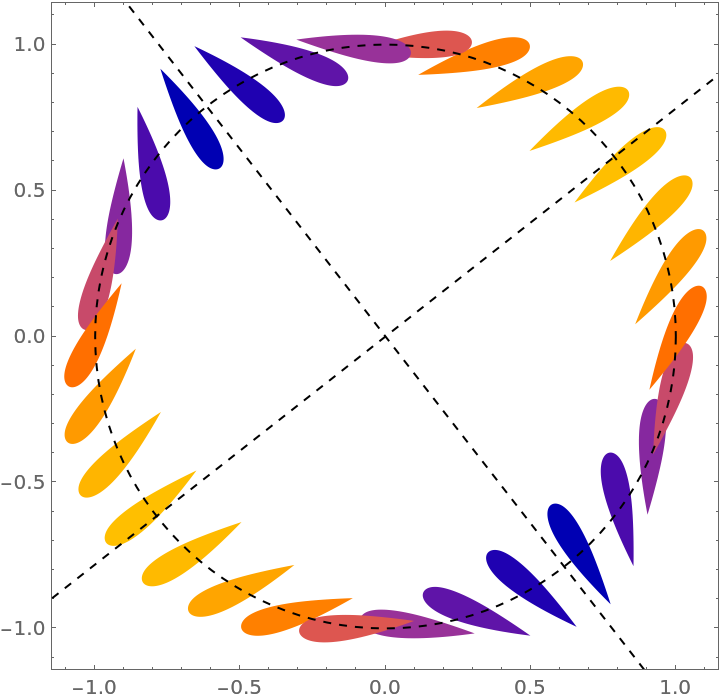The VectorPoints option can only take the values Automatic, Coarse, Fine or an integer:

 In:=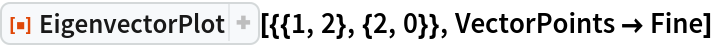Out=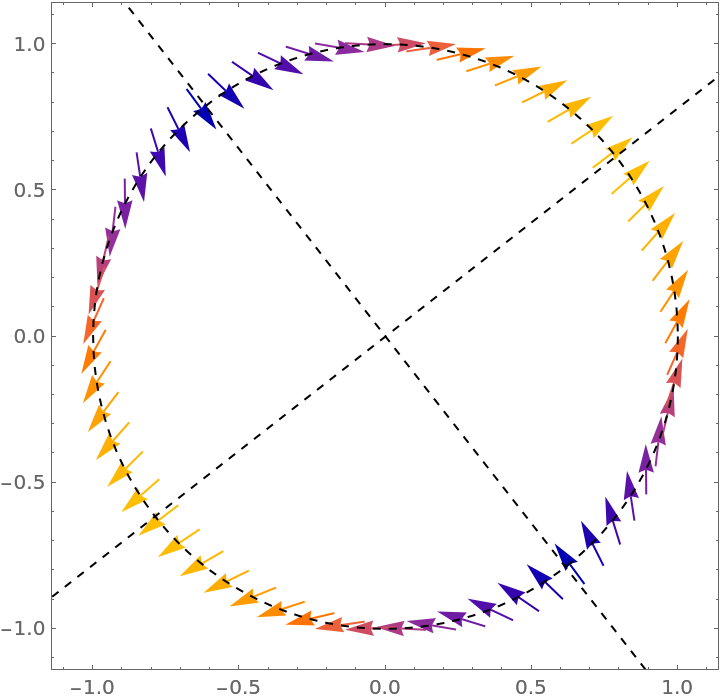In:=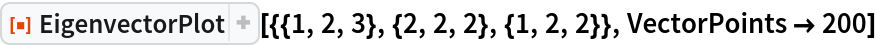Out=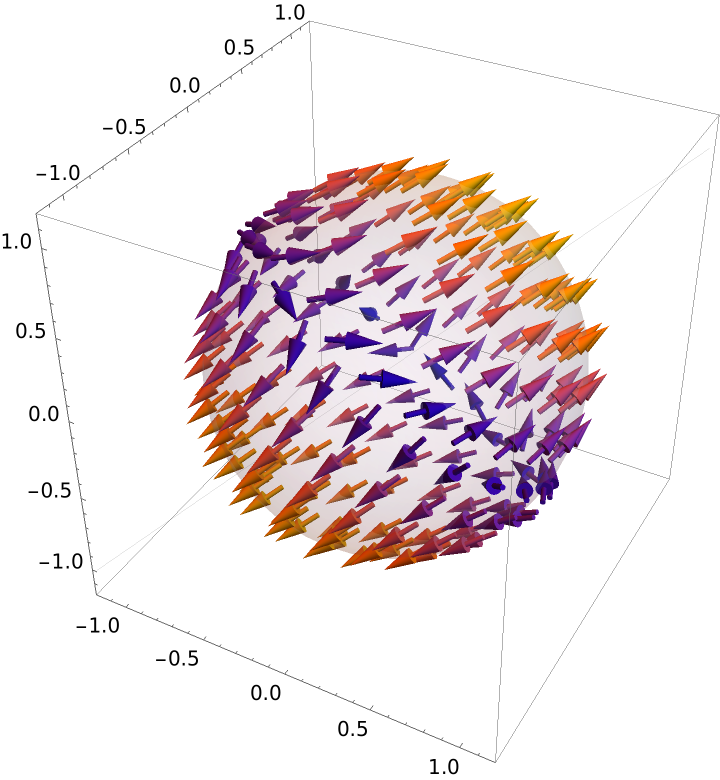Jon McLoone

## Version History

• 1.0.0 – 25 March 2020